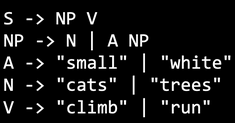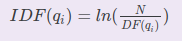# Quiz: Natural language processing¶

Consider the following context-free grammar, where S is the start symbol, and the four given sentences below.Can this sentence be derived from the context-free grammar?

“Cats run.”

Consider the following context-free grammar, where S is the start symbol, and the four given sentences below.Can this sentence be derived from the context-free grammar?

“Cats climb trees.”

Consider the following context-free grammar, where S is the start symbol, and the four given sentences below.Can this sentence be derived from the context-free grammar?

“Small cats run.”

Consider the following context-free grammar, where S is the start symbol, and the four given sentences below.Can this sentence be derived from the context-free grammar?

“Small white cats climb.”

Consider the phrase “must be the truth”. How many 2-grams can be extracted from it?

Consider the following corpus:

1. START Sam I am STOP

2. START I am Sam STOP

3. START I do not like green eggs and ham STOP

Using the bigram language model, calculate P(START Sam STOP). Round your answer to three decimal places and use a period as a decimal separator.

Consider the following corpus:

1. START Sam I am STOP

2. START I am Sam STOP

3. START I do not like green eggs and ham STOP

Using the bigram language model, calculate P(START Sam I like eggs STOP). Round your answer to two decimal places and use a period as a decimal separator.

Consider the following corpus:

1. START Sam I am STOP

2. START I am Sam STOP

3. START I do not like green eggs and ham STOP

Using the bigram language model, what is the perplexity of P(START Sam STOP)? Round your answer to two decimal places and use a period as a decimal separator.

Consider the following corpus consisting of 4 documents.

1. a a b c

2. a c c c d e f

3. a c d d d

4. a d f

What is the tf-idf value for “d” in Document 3? Round your answer to two decimal places and use a period as a decimal separator.

Use the following equation to calculate the inverse document frequency:where ln is the natural logarithm (log e )

Posting submission...